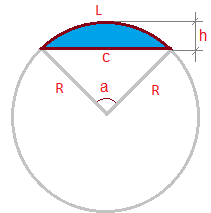homechevron_rightStudychevron_rightMathchevron_rightGeometry

# Arc length calculator

This univeral online calculator can find arc length of circular segment by radius and angle, by chord and height and by radius and height.

This online calculator computes the arc length of circular segment, given either the radius and angle of the segment, or the chord length and the height of the segment, or the radius and the height of the segment. Most useful thing, in my opinion, is the ability to find the arc length by the chord length and the height - these often can be measured directly (see the picture - the blue part is the circular segment, those arc length should be found).The circular segment

In the calculator below, choose the data you have to find the arc length, enter them and get the result. All formulas used for calculations are listed below the calculator.#### Arc length calculator

°
Digits after the decimal point: 2
Arc length

### Finding the arc length by the radius and the angle of the circular segment

The formula is simple

### Finding the arc length by the chord length and the height of the circular segment

Here you just need to calculate the radius and the angle, and then use the formula above.

The radius:

The angle:

### Finding the arc length by the radius and the height of the circular segment

Here you need to calculate the angle, then again use the formula

The angle:

For more universal calculator regarding circular segment in general, check out the Circular segment calculator. It finds chord length, segment height, segment perimeter, segment area and arc length, depending on what data do you have.